2.9.4. Dynamical friction

Once the collapse of a cluster is completed, violent relaxation is ineffective, and further relaxation occurs through two-body interactions. As shown above, these effects are probably not important for a typical galaxy at an average position in the cluster, but they can be significant for a more massive galaxy (m >> m*) in the cluster core (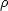>> <>; see equation 2.30). Chandrasekhar (1942) showed that a massive object of mass m moving at velocity v through a homogeneous, isotropic, Maxwellian distribution of lighter, collisionless particles suffers a drag force given by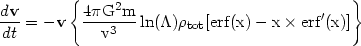(2.34)

where xv/(21/2r),r is the radial velocity dispersion of the lighter particles,tot is their total mass density, erf is the error function, andis given by equation (2.29). Note that this 'dynamical friction' force is independent of the mass of the lighter particles, and thus depends only on the total densitytot of such particles (regardless of whether they are galaxies or collisionless missing mass particles).

The relaxation time in the cluster core can be evaluated by noting that, for an isothermal cluster distribution (Section 2.7), the central density0, velocity dispersionr, and core radius rc are related by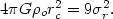(2.35)

Let m = (m/m*)m* = (m/m*)(M/LV)gal LV*, where LV* and m* are the characteristic visual luminosity and corresponding mass of galaxies in the Schechter luminosity function (Schechter, 1976, and Section 2.4), and M/LV is the visual mass-to-light ratio. Schechter finds LV*4.9 × 1010 L. Substituting this value, ln3, and the0 from equation (2.35) into equation (2.28) gives for the dynamical friction time-scale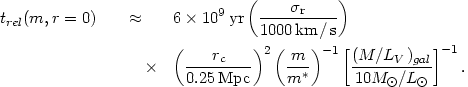(2.36)

Dynamical friction slows down the more massive galaxies near the center of a spherical cluster; they spiral in toward the cluster center (Lecar, 1975). The kinetic energy removed from the massive galaxies is transferred to the lighter particles (galaxies or missing mass components), which then expand. Thus dynamical friction produces mass segregation in a cluster; more massive galaxies are found preferentially at smaller radii. Moreover, at a fixed radius the velocity dispersion of the more massive galaxies will be lower, as they have been slowed down.

If the cluster is aspherical because of an anisotropic velocity dispersion, the dynamical friction force will not be parallel to the velocity (Binney, 1977). Because the force depends inversely on velocity, it is strongest along the shortest axis of the cluster. Thus dynamical friction increases the anisotropy of the most massive galaxies in a cluster. This can explain the formation of L clusters such as Perseus, in which the brightest galaxies form a narrow chain along the long axis of the cluster (Binney, 1977).

The massive galaxies that spiral into the cluster center will eventually merge to form a single supergiant galaxy if they are not tidally disrupted first. In Section 2.10.1 evidence is given which suggests that this is the mechanism by which cD galaxies form; however, there are also strong arguments that galaxies lose mass due to tidal effects so rapidly that dynamical friction is not important (Merritt, 1983, 1984a, 1985). The merger of the most massive and therefore brightest galaxies in the cluster core might cause the luminosity function to fall off more rapidly at high luminosities, as may have been observed in some cD clusters (Dressler, 1978b), and might produce a deficiency of brighter galaxies (other than the cD) in the cluster core (White, 1976a).

The fact that the dynamical friction time scale depends inversely on galaxy mass provides a method to measure the mass bound to individual galaxies in a cluster, as opposed to the total virial mass of the cluster. If the mass-to-light ratio derived for the cluster as a whole (typically (M/LV)250 M/ L; equation (2.25)) is substituted in equation (2.36), trel2 × 108 yr(m / m*)-1, which is much smaller than the probable age of a cluster of about a Hubble time th1010 yr (for typical parameters for a compact, regular cluster). Thus, if the missing mass in clusters were bound to individual galaxies, the massive galaxies m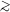m* would be highly relaxed (at least in the cluster core). All of these galaxies would have segregated into a very small core and possibly merged to form a single galaxy. Observations of the degree of mass segregation, luminosity function, and luminosity of the brightest galaxies in well studied clusters are all inconsistent with this much relaxation. From the observations one can limit the mass-to-light ratios of massive galaxies in cluster cores to (M/LV)30 M/ L. This indicates that the missing mass cannot be bound to individual, massive galaxies, but rather must form a continuum. This important result was first given by Rood (1965), and has been verified by numerical integration of equation (2.34) and N-body models by White (1976a, 1977b) and Merritt (1983).